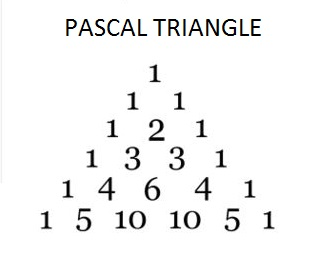# C Program to Print Pascal Triangle till N Rows

Here is a C program to print pascal triangle till N rows by calculating binomial coefficients.#### Required Knowledge

Pascal Triangle is a regular triangle of binomial coefficients. The counting of rows of the pascal triangle starts with 0 from top to bottom and elements in any particular row are numbered from left to right starting from 0.
Here is the formulae to find the value of nth element of rth row of pascal triangle.## C program to print pascal triangle till N rows

```#include <stdio.h>

int getFactorial(int n);

int main() {
int row, rows, i, value;

printf("Enter Number of Rows of Pascal Triangle\n");
scanf("%d", &rows);

for(row = 0; row < rows; row++) {
/* Print Spaces for every row */
for(i = row; i <= rows; i++)
printf("  ");

for(i = 0; i <= row; i++) {
value = getFactorial(row)/(getFactorial(i)*getFactorial(row-i));
printf("%4d", value);
}

printf("\n");
}

return 0;
}

int getFactorial(int N){
if(N < 0){
printf("Invalid Input: factorial not defined for \
negative numbers\n");
return 0;
}
int nFactorial = 1, counter;
/*  N! = N*(N-1)*(N-2)*(N-3)*.....*3*2*1  */
for(counter = 1; counter <= N; counter++){
nFactorial = nFactorial * counter;
}
return nFactorial;
}
```
Output
```Enter Number of Rows of Pascal Triangle
5
1
1 1
1 2 1
1 3 3 1
1 4 6 4 1
```

Related Topics
 C Program to print Floyd's triangle C program to print triangle and pyramid patterns of star(*) C program to print right triangle star pattern C program to print reversed mirrored right triangle star pattern C program to print natural numbers in right triangle pattern C program to print palindrome triangle pattern C program to print binary numbers right triangle pyramid pattern C program to convert octal number to binary number system C star triangle pattern programs List of all C programs